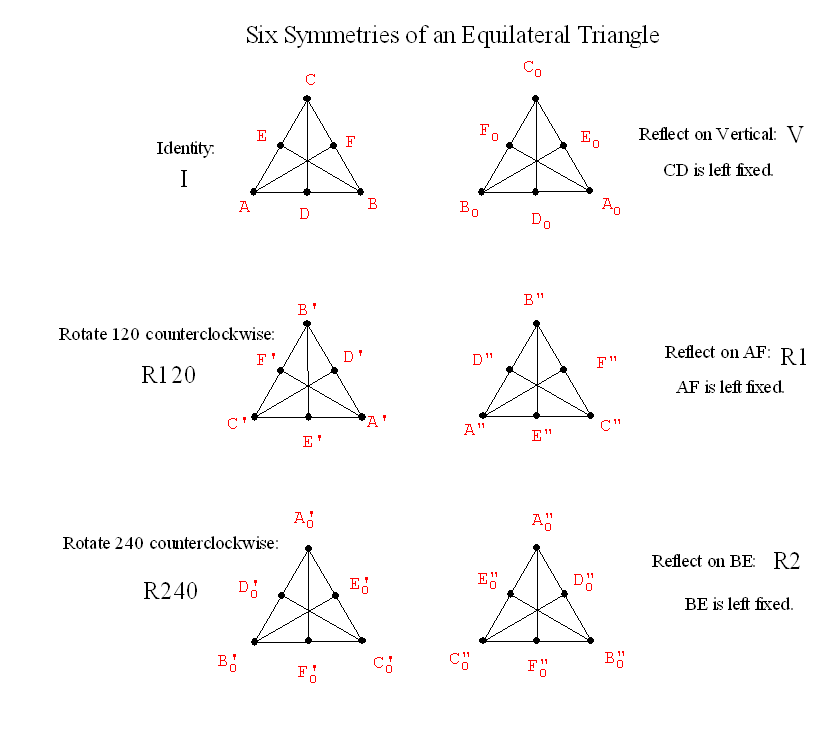Thursday,  February 10th

Reminder:
Project Proposals  and one (or two) portfolio entries should be submitted for preview feedback and advice by Thursday, February 25th.
Lab Tuesday Feb 15th SH 118. (for Wingeometry and other technology)

.
• Recall Symmetries of an equilateral triangle:• Combining transformations to give new symmetries:

Example: Let V denote reflection across the line that is the vertical altitude of the equilateral triangle.

And consider a second symmetry, called R=R120, which will rotate the equilateral triangle counterclockwise about its center O by 120 degrees.

We first perform V to the figure and then perform R to the figure.
We will denote this V*R... meaning V followed by R.

The resulting transformation also leaves the equilateral  covering the same position in which it started, and....

V*R     =   R2.

What about other products?  This gives a  "product" for symmetries.
If S and R are any symmetries of a figure then S*R is also a symmetry of the figure.

A "multiplication" table for Symmetries:
 * Id R120 R240 V G=R1 H=R2 Id I R120 R240 R1 R240 I R2 V R2 R1 I R120 G=R1 I H=R2 I
Do Activity.
This shows that R240*V = R1

This "multiplicative" structure  is called the Group of symmetries of the equilateral triangle.

Given any figure we can talk about the group of its symmetries.
Does a figure always have at least one symmetry? .....

Yes... The Identity symmetry.
Such a symmetry is called the trival symmetry.

So we can compare objects for symmetries....
how many?
does the multiplication table for the symmetries look the same in some sense?

Prelude for next week... Preview of coming attractions
Comparing figures:  The letters O and I have the same symmetries, but they are geometrically very different.
Compare  O  and 8. These have the same symmtries, but are geometrically very different.
Compare O and 0. These have the same symmetries but in many ways are not so different.
Find ways to distinguish O and 0 and ways they are comparable.
Initial discussion for projections and topological deformations and equivalences.

What about the symmetries of a Frieze Pattern on a Strip....
Translations and  Reflections

...|p|q|p|q|p|q|p|q|p|q|p|...

This pattern has vertical axes for reflective symmetries between each p and q... and a translation symmetry taking each letter to the next letter of the same type... and also twice as far, and three times as far , and more....

The same is true for the following pattern:

...|d|b|d|b|d|b|d|b|d|b|d|...

Notice the difference -180 degree Rotations and Translations are symmetries for the next pattern.
...|p|p|p|p|p|...
...|d|d|d|d|d|...

The following pattern also has glide reflection symmetry, for example taking p to b and q to d, etc.
It also has a 180 degree rotational symmetry with center midway between the vertical reflection axes and the letter p and d or q and b.

...|p|q|p|q|p|q|p|q|p|q|p|...
...|d|b|d|b|d|b|d|b|d|b|d|...

What are the possible symmetries of a Frieze Pattern on a Strip....

What about the symmetries of a tiling?

Based on the group of symmetries for these patterns,
there are seven possible distinct types of frieze or
Border Patterns:translationhorizontal reflectionvertical reflectionreflection + reflectionglide reflectionrotationreflection + glide reflection

Show FAPP Video on symmetry and patterns.

What about the symmetries of a
tiling? There are 17 distinct symmetry groups for tiling the plane. They can be described by the following diagrams indicating the symmetries of the figures  as below:or with figures as illustrated by the followingtranslationsreflectionsreflections + reflectionsglide reflectionsreflections + glide reflectionsrotations (2)reflections + rotations (2)rotations (2) + glide reflectionsrotations (2) + reflections + reflectionsrotations (4)reflections + rotations (4)rotations (4) + reflectionsrotations (3)reflections + rotations (3)rotations (3) + reflectionsrotations (6)reflections + rotations (6)

Do activity on recognizing symmetries in frieze and planar patterns.

One of the features of almost all we have done so far this term, the proofs of the Pythagorean Theorem,  Dissections, Tilings and Symmetry have involved
• Rigid Motions in (or about) the plane.  Also called "Isometries"
• Orientation preserving
• Translations
• Rotations
• Orientation reversing
• Reflections
• Glide reflections

• Classification of Isometries
Next Class...Video : Isometries
The video introduced the four isometries we have discussed:
reflections, rotations, translations, and glide reflections.

It was shown that the product of two reflections is either a rotation (if the axes of the reflection intersect)  or a translation (if the axes of the reflection are parallel).

Wingeometry demonstration for reflection- one and two reflections
What about 3 reflections?  How to figure out.... match features.

Every plane isometry is the product of at most three reflections.

Two reflections = rotation or translation.
• Three reflections = reflection or glide reflection

•
 Preserve Orientation Reverse Orientation No Fixed points Translation Glide reflection Fixed Point(s) Rotation Reflection
• Using Isometries to recognize symmetries of a figure or tiling.